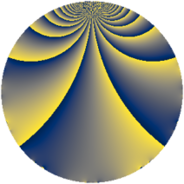# Properties

 Label 567.2.iLevel $567$ Weight $2$ Character orbit 567.i Rep. character $\chi_{567}(215,\cdot)$ Character field $\Q(\zeta_{6})$ Dimension $60$ Newform subspaces $7$ Sturm bound $144$ Trace bound $7$

# Learn more

## Defining parameters

 Level: $$N$$ $$=$$ $$567 = 3^{4} \cdot 7$$ Weight: $$k$$ $$=$$ $$2$$ Character orbit: $$[\chi]$$ $$=$$ 567.i (of order $$6$$ and degree $$2$$) Character conductor: $$\operatorname{cond}(\chi)$$ $$=$$ $$63$$ Character field: $$\Q(\zeta_{6})$$ Newform subspaces: $$7$$ Sturm bound: $$144$$ Trace bound: $$7$$ Distinguishing $$T_p$$: $$2$$, $$11$$, $$13$$

## Dimensions

The following table gives the dimensions of various subspaces of $$M_{2}(567, [\chi])$$.

Total New Old
Modular forms 168 68 100
Cusp forms 120 60 60
Eisenstein series 48 8 40

## Trace form

 $$60q - 52q^{4} + 7q^{7} + O(q^{10})$$ $$60q - 52q^{4} + 7q^{7} - 6q^{10} + 15q^{13} + 44q^{16} - 6q^{19} + 8q^{22} - 24q^{25} - 20q^{28} - 12q^{34} - 7q^{37} - 12q^{40} - 16q^{43} + 2q^{46} - 15q^{49} - 78q^{52} + 32q^{58} - 32q^{64} + 6q^{67} - 18q^{70} - 6q^{73} + 36q^{76} + 78q^{79} - 18q^{82} - 18q^{85} - 2q^{88} + 45q^{91} + 15q^{97} + O(q^{100})$$

## Decomposition of $$S_{2}^{\mathrm{new}}(567, [\chi])$$ into newform subspaces

Label Dim. $$A$$ Field CM Traces $q$-expansion
$$a_2$$ $$a_3$$ $$a_5$$ $$a_7$$
567.2.i.a $$2$$ $$4.528$$ $$\Q(\sqrt{-3})$$ $$\Q(\sqrt{-3})$$ $$0$$ $$0$$ $$0$$ $$-5$$ $$q+2q^{4}+(-2-\zeta_{6})q^{7}+(8-4\zeta_{6})q^{13}+\cdots$$
567.2.i.b $$2$$ $$4.528$$ $$\Q(\sqrt{-3})$$ $$\Q(\sqrt{-3})$$ $$0$$ $$0$$ $$0$$ $$4$$ $$q+2q^{4}+(1+2\zeta_{6})q^{7}+(2-\zeta_{6})q^{13}+\cdots$$
567.2.i.c $$4$$ $$4.528$$ $$\Q(\sqrt{-3}, \sqrt{-5})$$ None $$0$$ $$0$$ $$0$$ $$10$$ $$q+\beta _{3}q^{2}-3q^{4}+(2+\beta _{2})q^{7}-\beta _{3}q^{8}+\cdots$$
567.2.i.d $$4$$ $$4.528$$ $$\Q(\sqrt{-2}, \sqrt{-3})$$ None $$0$$ $$0$$ $$0$$ $$-8$$ $$q+\beta _{3}q^{2}+(\beta _{1}+\beta _{3})q^{5}+(-3+2\beta _{2}+\cdots)q^{7}+\cdots$$
567.2.i.e $$4$$ $$4.528$$ $$\Q(\sqrt{-2}, \sqrt{-3})$$ None $$0$$ $$0$$ $$0$$ $$-2$$ $$q+\beta _{3}q^{2}+(-\beta _{1}-\beta _{3})q^{5}+(1-3\beta _{2}+\cdots)q^{7}+\cdots$$
567.2.i.f $$12$$ $$4.528$$ $$\mathbb{Q}[x]/(x^{12} - \cdots)$$ None $$0$$ $$0$$ $$0$$ $$4$$ $$q-\beta _{11}q^{2}+(-1+\beta _{7})q^{4}-\beta _{6}q^{5}+\cdots$$
567.2.i.g $$32$$ $$4.528$$ None $$0$$ $$0$$ $$0$$ $$4$$

## Decomposition of $$S_{2}^{\mathrm{old}}(567, [\chi])$$ into lower level spaces

$$S_{2}^{\mathrm{old}}(567, [\chi]) \cong$$ $$S_{2}^{\mathrm{new}}(63, [\chi])$$$$^{\oplus 3}$$$$\oplus$$$$S_{2}^{\mathrm{new}}(189, [\chi])$$$$^{\oplus 2}$$